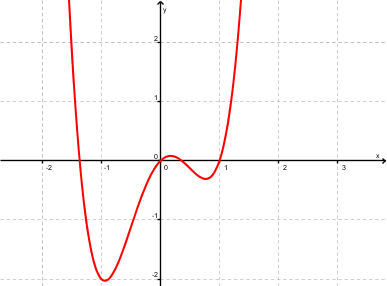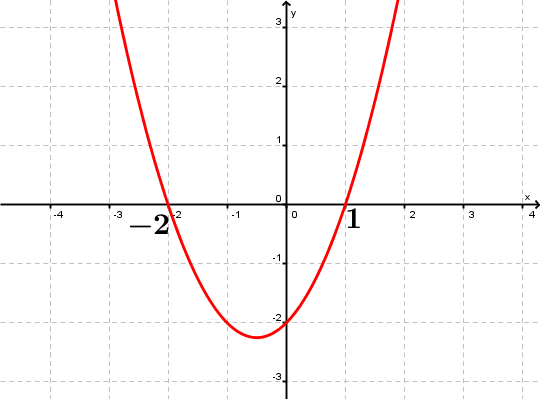Math Calculators, Lessons and Formulas

It is time to solve your math problem

mathportal.org
• Polynomials
• Roots of Polynomials
• Properties of polynomial roots

# Properties of polynomial roots

ans:
syntax error
C
DEL
ANS
±
(
)
÷
×
7
8
9
4
5
6
+
1
2
3
=
0
.
auto next question
evaluate answers
calculator
•  Question 1: 1 pts $x=3$ is a root of polynomial $p(x) = x^2-x-6$.
•  Question 2: 1 pts $x=-1$ is a root of polynomial $p(x) = x^3-x+2x+3$
•  Question 3: 1 pts The graph of the polynomial $p(x)$ is given at the right. How many positive roots does $p(x)$ has?0 1 2 3
•  Question 4: 2 pts How many distinct roots does polynomial $p(x) = (x+1)^2$ has
 $0$ $1$ $2$ $3$
•  Question 5: 2 pts One zero of $p(x) = x^2+2x+2$ is $1+i$. Which of the following is another zero?
 $i$ $i-1$ $1-i$ $-i$
•  Question 6: 2 pts One zero of $p(x) = x^3- 3x^2+ x + 5$ is $2 - i$. Which of the following is another zero?
 $2$ $2+i$ $i-2$ $i$
•  Question 7: 2 pts The equation of polynomial whose graph is shown in the figure is:$p(x)=(x+2)(x+1)$ $p(x)=(x-2)(x-1)$ $p(x)=(x+2)(x-1)$
•  Question 8: 2 pts Polynomial $p(x)$ has roots $x=1$ and $x=2$. $$p(x)=$$
 $x^2-2x+1$ $x^2+x-4$ $x^2-3x+2$ none of these
•  Question 9: 3 pts Polynomial $p(x)$ has roots $x=1$ , $x=5$ and $x=0$. $$p(x) =$$
 $x^3-5x^2+6x$ $x^3-6x^2+5x$ $x^3-x^2$ none of these
•  Question 10: 2 pts Multiplicity of root $x=1$ in polynomial $p(x)=(x+1)^2$ is 2.
•  Question 11: 2 pts multiplicity of root $x=1$ in polynomial $p(x)=3(x-1)^2(x+1)^3$ is 3.
•  Question 12: 2 pts Polynomial $p(x)$, with leading coefficient 6, has a root of multiplicity 7 at $x=0$. The equation of polynomial $p(x)$ is $7x^6$.
•  Question 13: 3 pts Find cubic polynomial with real coefficients having roots $x = - 2$ and $x = i$.
 $x^3+2x^2+x+2$ $x^3+2x-4$ $2x^3-x+14$
•  Question 14: 3 pts Polynomial of degree 4, has a root of multiplicity 2 at $x=-1$ and roots of multiplicity 1 at $x=0$ and $x=4$? The equation of polynomial is:
 $x(x+4)(x-1)^2$ $x(x+4)(x+1)^2$ $x(x-4)(x+1)^2$ $x(x-4)(x-1)^2$